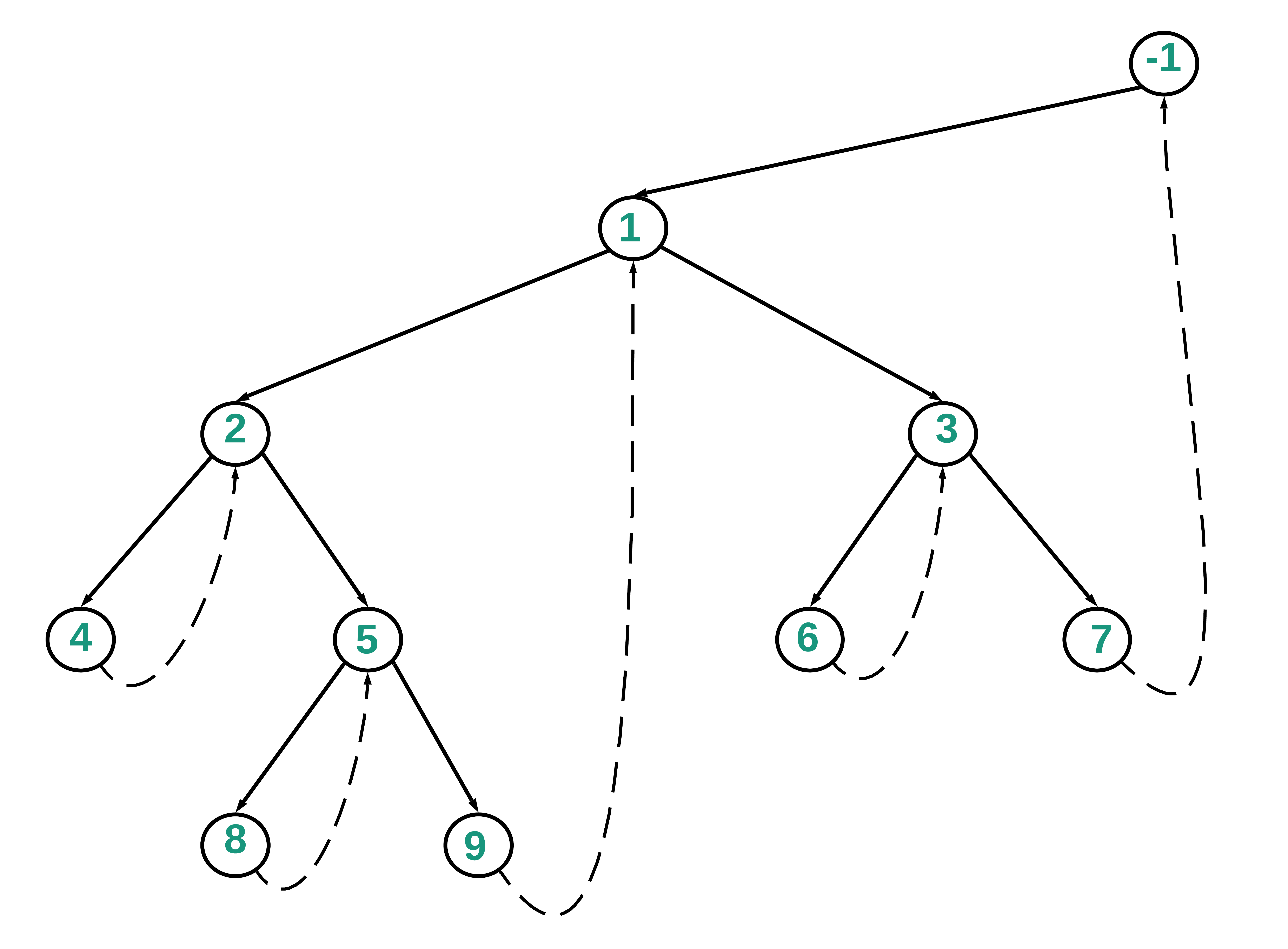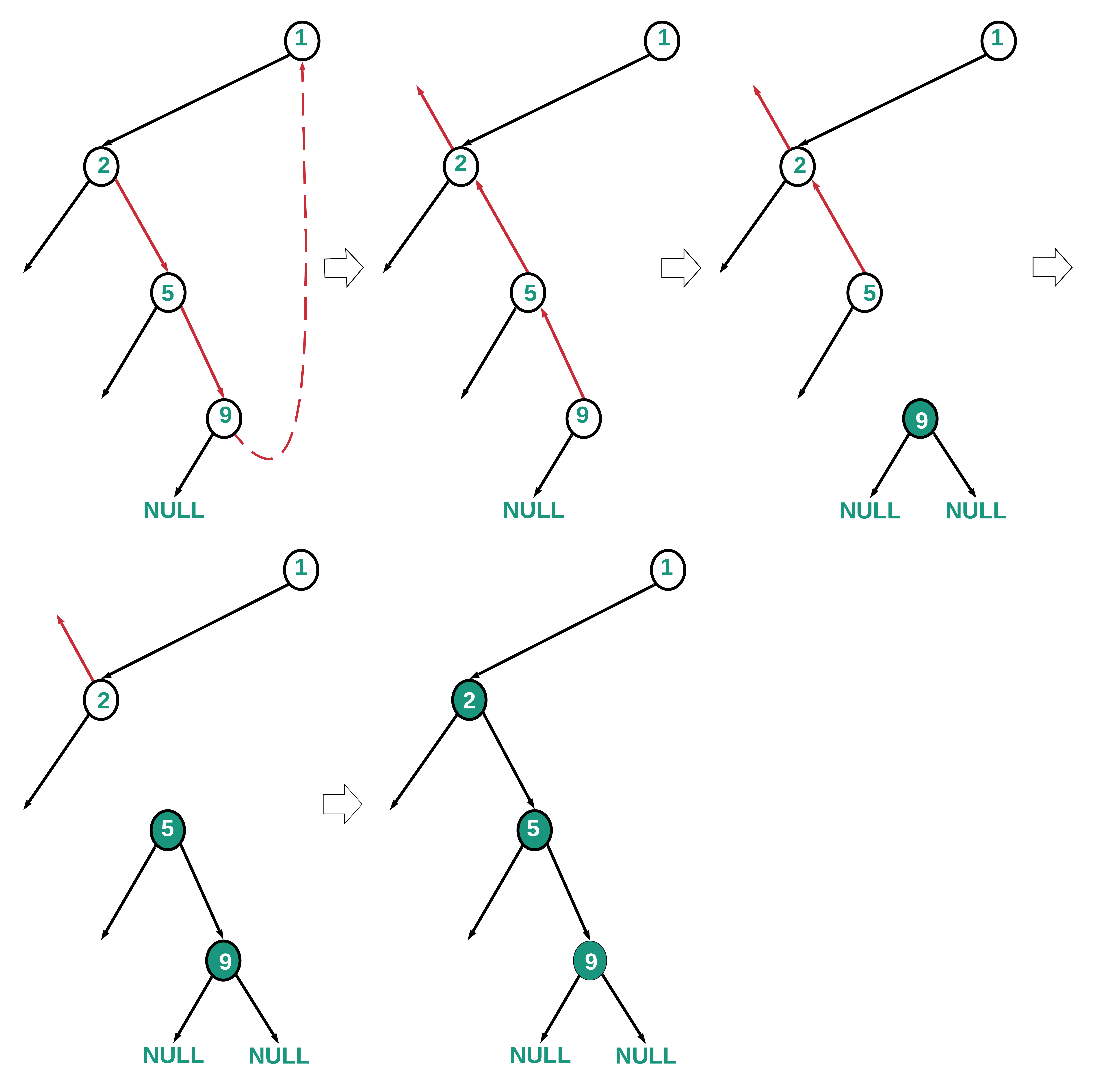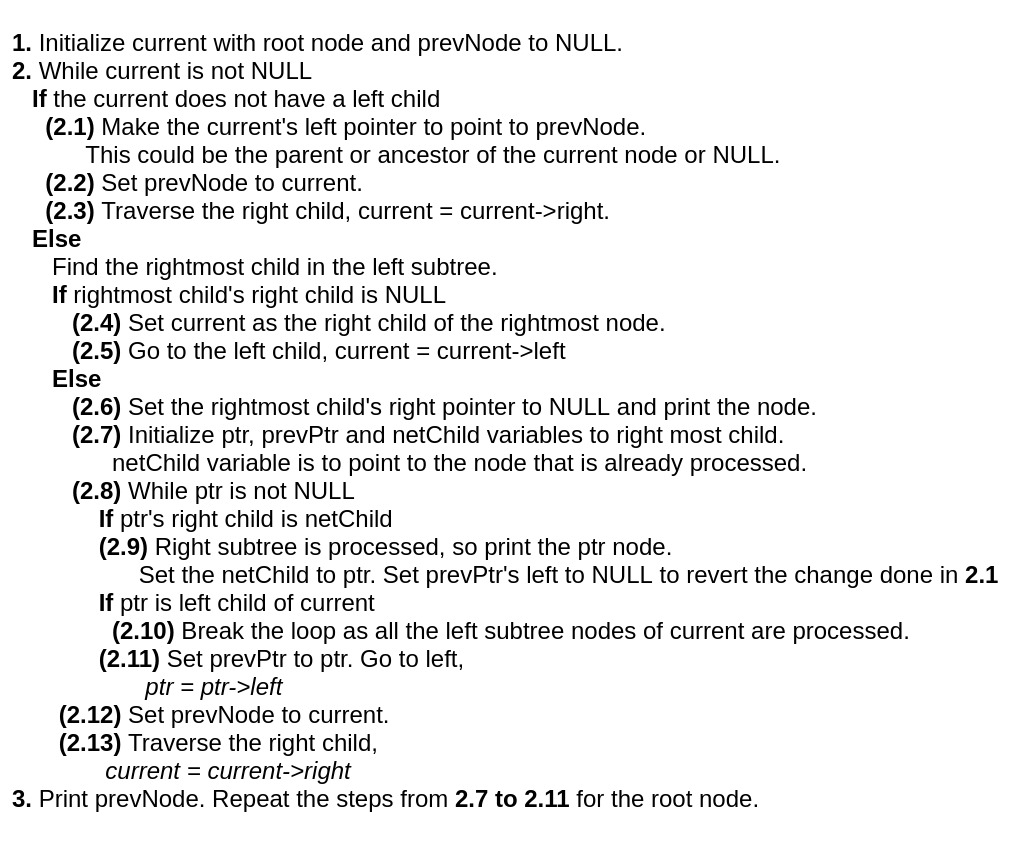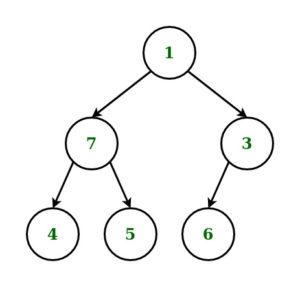1595376000

# Post Order Traversal of Binary Tree in O(N) using O(1) space

Given a Binary Tree, the task is to print the elements in post order using O(N) time complexity and constant space.

``````Input:   1
/   \
2       3
/ \     / \
4   5   6   7
/ \
8   9
Output: 4 8 9 5 2 6 7 3 1

Input:   5
/   \
7       3
/ \     / \
4   11  13  9
/ \
8   4
Output: 4 8 4 11 7 13 9 3 5
``````

## Recommended: Please try your approach on {IDE} first, before moving on to the solution.

Method 1: Using Morris Inorder Traversal

1. Create a dummy node and make the root as it’s left child.
2. Initialize current with dummy node.
3. While current is not NULL
• If current does not have a left chil traverse the right child, current = current->right
• Otherwise,
1. Find the right most child in the left subtree.
2. If right most child’s right child is NULL
• Make current as the right child of the right most node.
• Traverse the left child, current = current->left
1. Otherwise,
• Set the right most child’s right pointer to NULL.
• From current’s left child, traverse along the right children until the right most child and reverse the pointers.
• Traverse back from right most child to current’s left child node by reversing the pointers and printing the elements.
1. Traverse the right child, current = current->right

Below is the diagram showing the right most child in left subtree, pointing to it’s inorder successor.Below is the diagram which highlights the path 1->2->5->9 and the way the nodes are processed and printed as per the above algorithm.Below is the implementation of the above approach:

• Java

filter_none

edit

play_arrow

brightness_4

`// Java program to implement`

`// Post Order traversal`

`// of Binary Tree in O(N)`

`// time and O(1) space`

`// Definition of the`

`// binary tree`

`**class**` `TreeNode {`

`**public**` `**int**` `data;`

`**public**` `TreeNode left;`

`**public**` `TreeNode right;`

`**public**` `TreeNode(``**int**` `data)`

`{`

`**this**``.data = data;`

`}`

`**public**` `String toString()`

`{`

`**return**` `data +` `" "``;`

`}`

`}`

`**public**` `**class**` `PostOrder {`

`TreeNode root;`

`// Function to find Post Order`

`// Traversal Using Constant space`

`**void**` `postOrderConstantspace(TreeNode`

`root)`

`{`

`**if**` `(root ==` `**null**``)`

`**return**``;`

`TreeNode current`

`=` `**new**` `TreeNode(-``1``),`

`pre =` `**null**``;`

`TreeNode prev =` `**null**``,`

`succ =` `**null**``,`

`temp =` `**null**``;`

`current.left = root;`

`**while**` `(current !=` `**null**``) {`

`// Go to the right child`

`// if current does not`

`// have a left child`

`**if**` `(current.left ==` `**null**``) {`

`current = current.right;`

`}`

`**else**` `{`

`// Traverse left child`

`pre = current.left;`

`// Find the right most child`

`// in the left subtree`

`**while**` `(pre.right !=` `**null**`

`&& pre.right != current)`

`pre = pre.right;`

`**if**` `(pre.right ==` `**null**``) {`

`// Make current as the right`

`// child of the right most node`

`pre.right = current;`

`// Traverse the left child`

`current = current.left;`

`}`

`**else**` `{`

`pre.right =` `**null**``;`

`succ = current;`

`current = current.left;`

`prev =` `**null**``;`

`// Traverse along the right`

`// subtree to the`

`// right-most child`

`**while**` `(current !=` `**null**``) {`

`temp = current.right;`

`current.right = prev;`

`prev = current;`

`current = temp;`

`}`

`// Traverse back from`

`// right most child to`

`// current's left child node`

`**while**` `(prev !=` `**null**``) {`

`System.out.print(prev);`

`temp = prev.right;`

`prev.right = current;`

`current = prev;`

`prev = temp;`

`}`

`current = succ;`

`current = current.right;`

`}`

`}`

`}`

`}`

`// Driver Code`

`**public**` `**static**` `**void**` `main(String[] args)`

`{`

`/* Constructed tree is as follows:-`

`1 `

`/     \`

`2       3`

`/ \     / \`

`4   5   6   7`

`/ \`

`8   9`

`*/`

`PostOrder tree =` `**new**` `PostOrder();`

`tree.root =` `**new**` `TreeNode(``1``);`

`tree.root.left =` `**new**` `TreeNode(``2``);`

`tree.root.right =` `**new**` `TreeNode(``3``);`

`tree.root.left.left =` `**new**` `TreeNode(``4``);`

`tree.root.left.right`

`=` `**new**` `TreeNode(``5``);`

`tree.root.right.left`

`=` `**new**` `TreeNode(``6``);`

`tree.root.right.right`

`=` `**new**` `TreeNode(``7``);`

`tree.root.left.right.left`

`=` `**new**` `TreeNode(``8``);`

`tree.root.left.right.right`

`=` `**new**` `TreeNode(``9``);`

`tree.postOrderConstantspace(`

`tree.root);`

`}`

`}`

Output:

``````4 8 9 5 2 6 7 3 1
``````

_Time Complexity: _O(N)

Auxiliary Space:_ O(1)_

Method 2: In method 1, we traverse a path, reverse references, print nodes as we restore the references by reversing them again. In method 2, instead of reversing paths and restoring the structure, we traverse to parent node from the current node using the current node’s left subtree. This could be faster depending on the tree structure, for example in a right-skewed tree.

The following algorithm and diagrams provide the details of the approach.#data structures #recursion #tree #inorder traversal #interview-preparation #morris-traversal #postorder traversal

## Buddha Community1595376000

## Post Order Traversal of Binary Tree in O(N) using O(1) space

Given a Binary Tree, the task is to print the elements in post order using O(N) time complexity and constant space.

``````Input:   1
/   \
2       3
/ \     / \
4   5   6   7
/ \
8   9
Output: 4 8 9 5 2 6 7 3 1

Input:   5
/   \
7       3
/ \     / \
4   11  13  9
/ \
8   4
Output: 4 8 4 11 7 13 9 3 5
``````

## Recommended: Please try your approach on {IDE} first, before moving on to the solution.

Method 1: Using Morris Inorder Traversal

1. Create a dummy node and make the root as it’s left child.
2. Initialize current with dummy node.
3. While current is not NULL
• If current does not have a left chil traverse the right child, current = current->right
• Otherwise,
1. Find the right most child in the left subtree.
2. If right most child’s right child is NULL
• Make current as the right child of the right most node.
• Traverse the left child, current = current->left
1. Otherwise,
• Set the right most child’s right pointer to NULL.
• From current’s left child, traverse along the right children until the right most child and reverse the pointers.
• Traverse back from right most child to current’s left child node by reversing the pointers and printing the elements.
1. Traverse the right child, current = current->right

Below is the diagram showing the right most child in left subtree, pointing to it’s inorder successor.Below is the diagram which highlights the path 1->2->5->9 and the way the nodes are processed and printed as per the above algorithm.Below is the implementation of the above approach:

• Java

filter_none

edit

play_arrow

brightness_4

`// Java program to implement`

`// Post Order traversal`

`// of Binary Tree in O(N)`

`// time and O(1) space`

`// Definition of the`

`// binary tree`

`**class**` `TreeNode {`

`**public**` `**int**` `data;`

`**public**` `TreeNode left;`

`**public**` `TreeNode right;`

`**public**` `TreeNode(``**int**` `data)`

`{`

`**this**``.data = data;`

`}`

`**public**` `String toString()`

`{`

`**return**` `data +` `" "``;`

`}`

`}`

`**public**` `**class**` `PostOrder {`

`TreeNode root;`

`// Function to find Post Order`

`// Traversal Using Constant space`

`**void**` `postOrderConstantspace(TreeNode`

`root)`

`{`

`**if**` `(root ==` `**null**``)`

`**return**``;`

`TreeNode current`

`=` `**new**` `TreeNode(-``1``),`

`pre =` `**null**``;`

`TreeNode prev =` `**null**``,`

`succ =` `**null**``,`

`temp =` `**null**``;`

`current.left = root;`

`**while**` `(current !=` `**null**``) {`

`// Go to the right child`

`// if current does not`

`// have a left child`

`**if**` `(current.left ==` `**null**``) {`

`current = current.right;`

`}`

`**else**` `{`

`// Traverse left child`

`pre = current.left;`

`// Find the right most child`

`// in the left subtree`

`**while**` `(pre.right !=` `**null**`

`&& pre.right != current)`

`pre = pre.right;`

`**if**` `(pre.right ==` `**null**``) {`

`// Make current as the right`

`// child of the right most node`

`pre.right = current;`

`// Traverse the left child`

`current = current.left;`

`}`

`**else**` `{`

`pre.right =` `**null**``;`

`succ = current;`

`current = current.left;`

`prev =` `**null**``;`

`// Traverse along the right`

`// subtree to the`

`// right-most child`

`**while**` `(current !=` `**null**``) {`

`temp = current.right;`

`current.right = prev;`

`prev = current;`

`current = temp;`

`}`

`// Traverse back from`

`// right most child to`

`// current's left child node`

`**while**` `(prev !=` `**null**``) {`

`System.out.print(prev);`

`temp = prev.right;`

`prev.right = current;`

`current = prev;`

`prev = temp;`

`}`

`current = succ;`

`current = current.right;`

`}`

`}`

`}`

`}`

`// Driver Code`

`**public**` `**static**` `**void**` `main(String[] args)`

`{`

`/* Constructed tree is as follows:-`

`1 `

`/     \`

`2       3`

`/ \     / \`

`4   5   6   7`

`/ \`

`8   9`

`*/`

`PostOrder tree =` `**new**` `PostOrder();`

`tree.root =` `**new**` `TreeNode(``1``);`

`tree.root.left =` `**new**` `TreeNode(``2``);`

`tree.root.right =` `**new**` `TreeNode(``3``);`

`tree.root.left.left =` `**new**` `TreeNode(``4``);`

`tree.root.left.right`

`=` `**new**` `TreeNode(``5``);`

`tree.root.right.left`

`=` `**new**` `TreeNode(``6``);`

`tree.root.right.right`

`=` `**new**` `TreeNode(``7``);`

`tree.root.left.right.left`

`=` `**new**` `TreeNode(``8``);`

`tree.root.left.right.right`

`=` `**new**` `TreeNode(``9``);`

`tree.postOrderConstantspace(`

`tree.root);`

`}`

`}`

Output:

``````4 8 9 5 2 6 7 3 1
``````

_Time Complexity: _O(N)

Auxiliary Space:_ O(1)_

Method 2: In method 1, we traverse a path, reverse references, print nodes as we restore the references by reversing them again. In method 2, instead of reversing paths and restoring the structure, we traverse to parent node from the current node using the current node’s left subtree. This could be faster depending on the tree structure, for example in a right-skewed tree.

The following algorithm and diagrams provide the details of the approach.#data structures #recursion #tree #inorder traversal #interview-preparation #morris-traversal #postorder traversal1598364000

## Mix Order Traversal of a Binary Tree

Given a Binary Tree consisting of** N** nodes, the task is to print its Mix Order Traversal.

Mix Order Traversal_ is a tree traversal technique, which involves any two of the existing traversal techniques like Inorder, Preorder and Postorder Traversal. Any two of them can be performed or alternate levels of given tree and a mix traversal can be obtained._

Examples:

_Input: _N = 6_Output: __7 4 5 1 3 6 _

Explanation:

_Inorder-Preorder Mix Traversal is applied to the given tree in the following order: _

_Inorder Traversal is applied at level 0 _

_Preorder Traversal is applied at level 1 _

Inorder Traversal at level 2._Output: __4 5 7 1 6 3 _

#data structures #recursion #tree #inorder traversal #postorder traversal #preorder traversal #tree traversals1599043260

## Check if all the Nodes in a Binary Tree having common values are at least D distance apart

Given a Binary Tree and an integer D, the task is to check if the distance between all pairs of same node values in the Tree is ? D or not. If found to be true, then print Yes. Otherwise, print No.

Examples:

Input:_ D = 7 _

``````                1
/   \
2     3
/ \   /  \
4   3  4   4

``````

Output:_ Yes _

Explanation:

_The repeated value of nodes are 3 and 4. _

_The distance between the two nodes valued 3, is 3. _

_The maximum distance between any pair of nodes valued 4 is 4. _

Therefore, none of the distances exceed 7

Input:_ D = 1 _

``````          3
/ \
3   3
\
3

``````

Output:_ No _

Recommended: Please try your approach on {IDE} first, before moving on to the solution.

**Approach: **

The idea is to observe that the problem is similar to finding the distance between two nodes of a tree. But there can be multiple pairs of nodes for which we have to find the distance. Follow the steps below:

1. Perform the Post Order Traversal of the given tree and find the distance between the repeated pairs of nodes.
2. Find the nodes that are repeated in the tree using unordered_map.
3. For each repeated node of a particular value, find the maximum possible distance between any pair.
4. If that distance is > D, print “No”.
5. If no such node value is found having a pair containing that value, exceeding **D, **then print “Yes”.

#greedy #recursion #searching #tree #binary tree #frequency-counting #postorder traversal #tree-traversal1598184540

## Double Order Traversal of a Binary Tree

Given a Binary Tree consisting of** N** nodes, the task is to print its Double Order Traversal.

Double Order Traversal_ is a tree traversal technique in which every node is traversed twice in the following order: _

• Visit the Node.
• Traverse the Left Subtree.
• Visit the Node.
• Traverse the Right Subtree.

Examples:

``````Input:
1
/   \
7     3
/ \   /
4   5 6
Output: 1 7 4 4 7 5 5 1 3 6 6 3

Input:
1
/   \
7     3
/ \     \
4   5     6
Output: 1 7 4 4 7 5 5 1 3 3 6 6

``````

Recommended: Please try your approach on {IDE} first, before moving on to the solution.

Approach:

The idea is to perform Inorder Traversal recursively on the given Binary Tree and print the node value on **visiting a vertex **and after the recursive call to the left subtree during the traversal.

Follow the steps below to solve the problem:

#data structures #recursion #tree #binary tree #inorder traversal #data analysis1598227320

## Remove all leaf nodes from a Generic Tree or N-ary Tree

Given a Generic tree, the task is to delete the leaf nodes from the tree.

** Examples:**

``````Input:
5
/  /  \  \
1   2   3   8
/   / \   \
15  4   5   6

Output:
5 : 1 2 3
1 :
2 :
3 :

Explanation:
Deleted leafs are:
8, 15, 4, 5, 6

Input:
8
/    |    \
9      7       2
/ | \    |    / / | \ \
4  5 6    10  11 1 2  2 3
Output:
8: 9 7 2
9:
7:
2:
``````

**Approach: **Follow the steps given below to solve the problem

• Take tree into the vector.
• Traverse the tree and check the condition:
• If current node is leaf then
• Delete the leaf from vector
• Else
• Recursively call for every child.

Below is the implementation of the above approach:

#data structures #recursion #tree #n-ary-tree #tree-traversal #data analysis